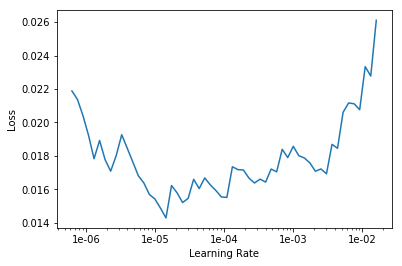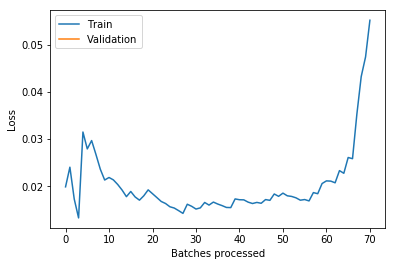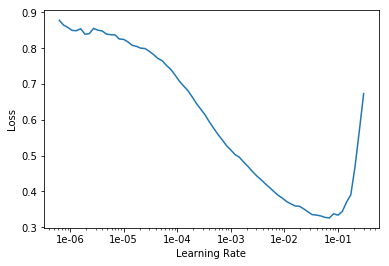# Poor cats and dogs submission using resnet

hi all, i am new to fastai and I experimented with Cats and Dogs dataset after watching Lesson 1.
I am using fastai version 1.0.52 . I have downloaded data from kaggle on colab and arranged data in “path” folder having folders “train’, “test” and"valid” with labelled folders in both “train” and “valid” folders(i.e. cats and dogs). The following is the code I have used

``````from fastai.vision import *
from fastai.metrics import error_rate
np.random.seed(0)
data = (ImageList.from_folder('path/train')
.split_by_rand_pct()
.label_from_folder()
.transform(get_transforms(),size=224)
.databunch(bs=64).normalize(imagenet_stats))
model=cnn_learner(data,models.resnet50,metrics=error_rate)
model.fit_one_cycle(5)
model.lr_find()
model.recorder.plot()
``````

got the following graph``````model.unfreeze()
model.fit_one_cycle(3,max_lr=slice(1e-6,1e-5))
model.export()
defaults.device = torch.device('cpu')
test=(ImageList.from_folder('path/test'))
log_preds_test= learn.get_preds(ds_type=DatasetType.Test)
``````

after that saved the results in a .csv file

``````labelled_preds = [float(max(pred,pred)) for pred in log_preds_test]
fnames = [f.name[:-4] for f in learn.data.test_ds.items]
fnames=list(map(int,fnames))
df = pd.DataFrame({'id':fnames, 'label':labelled_preds}, columns=['id', 'label'])

df=df.sort_values(by=['id'])
df.to_csv('path/submission.csv', index=False)
``````

made a few submissions in kaggle and got score of 3.015,3.7,4.755…which are terrible regarding the best scores to be 0.033-0.04.
Can anyone kindly show me any mistakes that I might have done?

Could you also provide all learning output including losses and metric?

this is the result after training the last layers of pretrained model

epoch train_loss valid_loss error_rate time
0 0.069317 0.040504 0.014750 03:37
1 0.052036 0.024831 0.009000 03:38
2 0.038391 0.017498 0.006500 03:37
3 0.026468 0.017167 0.007750 03:36
4 0.021890 0.021624 0.007750 03:37

and this is after i unfreeze the whole model

epoch train_loss valid_loss error_rate time
0 0.022924 0.016856 0.005250 03:52
1 0.020853 0.016505 0.006250 03:52
2 0.016733 0.016715 0.006000 03:51

Which learning rate did you use for phase 1 learning? Can you show the plot of the learning rate scheduler?

Also I recommend to add a callback to save the best model constrained on the metrics to safeguard against overfitting, which in your case seems to have happened.is this the one you are asking?

for phase 1 , i did not specify any learning rate, and can you give me more details how you observed my model has overfitted?

Regarding overfitting take a read here:

Regarding learning rate, after performing model.lr_find() please execute model.recorder.plot() and show what it tells. Based on that you should choose a learning rate.

All this is also demonstrated in the video lessons of course 1.

I have already attached the result of model.recorder.plot() in my question , and that’s why I set learning rate as slice(1e-6,1e-5) after unfreezing the model. Still got bad results

You did not attach this before phase 1. That may be more important. Look at the original notebook and try to reproduce these steps.

can you clarify what you mean by phase 1?.. is this before the unfreezing step or something elseI think this is the plot you were referring to

Exactly. Based on that replace

with e.g.

model.fit_one_cycle(5, 3e-4)

`model.fit_one_cycle(5,max_lr=3e-4)`

got output

epoch train_loss valid_loss error_rate time
0 0.022043 0.020784 0.009500 03:44
1 0.026131 0.018442 0.007000 03:42
2 0.024615 0.016825 0.006250 03:45
3 0.017526 0.017247 0.005500 03:44
4 0.021449 0.017330 0.005250 03:45

then ran this code

``````model.unfreeze()
model.fit_one_cycle(3,max_lr=slice(5e-6,1e-4))
``````

with output

epoch train_loss valid_loss error_rate time
0 0.022310 0.021581 0.007750 03:58
1 0.023473 0.020907 0.008250 03:54
2 0.009675 0.019023 0.006250 03:54

finally executed the test dataset and submitted to kaggle , unfortunately got a score of 5.7196 , is there still something I am missing?

Can you post which exact competition it is?

Its the Dogs vs. Cats Redux: Kernels Edition on kaggle

I just found that others have the same problem. See here:

Not obvious what is going on, sorry.

okay no problem… can you throw me some light on the heatmap type visuals that I can see when i plot top losses?

Considering that this competition uses the cross entropy loss as the target metric, what you are seeing here is your model being very accurate, but also very confident about its wrong predictions. It is also likely that the validation set is not particularly similar to the test set, so that throws you off.

You probably want to consider doing TTA

1 Like

hi , I applied TTA and submitted results on kaggle, got a score of 5.14650

The submission file should contain the probabilities that the image is a dog: https://www.kaggle.com/c/dogs-vs-cats-redux-kernels-edition/overview/evaluation. But you are submitting `labelled_preds = [float(max(pred,pred)) for pred in log_preds_test]` which is incorrect. You need to submit `labelled_preds = log_preds_test[:, 0(or 1)].numpy()` depending upon the index of the dog class. Also, verify the order of the predictions from the sample_submission_file in case you still get a bad score.

3 Likes

@rohit_gr you were absolutely correct, it was a terrible mistake on my part, got a score of 0.06033thank you very much and thank you @miko for suggesting to use TTA

1 Like